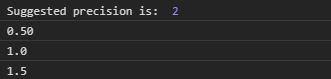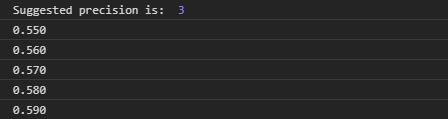# D3.js precisionRound() Function

• Last Updated : 29 Jul, 2020

The precisionRound() function of D3.js is used to calculate the significant digits that have to be after the decimal for rounded notation. It takes two parameters the step and max.

Syntax:

Hey geek! The constant emerging technologies in the world of web development always keeps the excitement for this subject through the roof. But before you tackle the big projects, we suggest you start by learning the basics. Kickstart your web development journey by learning JS concepts with our JavaScript Course. Now at it's lowest price ever!

```d3.precisionRound(step, max);
```

Parameters: It takes the two parameters as mentioned above and described below:

• Step: It tells the minimum absolute difference between two values that need to be formatted.
• Max: It denotes the maximum absolute value that is needed to be formatted.

Returns: It returns the Number.

Example 1:

## HTML

 ```<``html` `lang``=``"en"``>`` ` `<``head``>``    ``<``meta` `charset``=``"UTF-8"``>``    ``<``meta` `name``=``"viewport"` `content``=``        ``"width=device-width, initial-scale=1.0"``>```` ` `<``body``>``    ````    ``<``script` `type``=``"text/javascript"` `        ``src``=``"https://d3js.org/d3.v4.min.js"``>``    ````     ` `    ``<``script``>``        ``var p = d3.precisionRound(0.5, 1.5);``     ` `        ``// For .5, 1.0, 1.5 the step should``        ``// be 0.5 And the max should be 1.5``        ``// so suggested precision is 2``        ``console.log("Suggested precision is: ", p)``        ``var f = d3.format("." + p + "r");``        ``console.log(f(.5));``        ``console.log(f(1.0));``        ``console.log(f(1.5));``    `````` ` ``

Output:Example 2:

## HTML

 ```<``html` `lang``=``"en"``>`` ` `<``head``>``    ``<``meta` `charset``=``"UTF-8"``>``    ``<``meta` `name``=``"viewport"` `content``=``        ``"width=device-width, initial-scale=1.0"``>```` ` `<``body``>``    ````    ``<``script` `type``=``"text/javascript"` `        ``src``=``"https://d3js.org/d3.v4.min.js"``>``    ````     ` `    ``<``script``>``        ``var p = d3.precisionRound(0.01, 1.01);``     ` `        ``// For .55, .56,... the step should``        ``// be 0.01 And the max should be 1.01``        ``// so suggested precision is 3``        ``console.log("Suggested precision is: ", p)``        ``var f = d3.format("." + p + "r");``        ``console.log(f(.55));``        ``console.log(f(0.56));``        ``console.log(f(0.57));``        ``console.log(f(.58));``        ``console.log(f(.59));``    `````` ` ``

Output:My Personal Notes arrow_drop_up